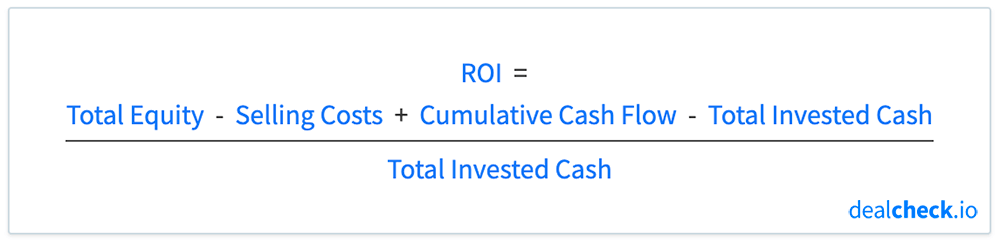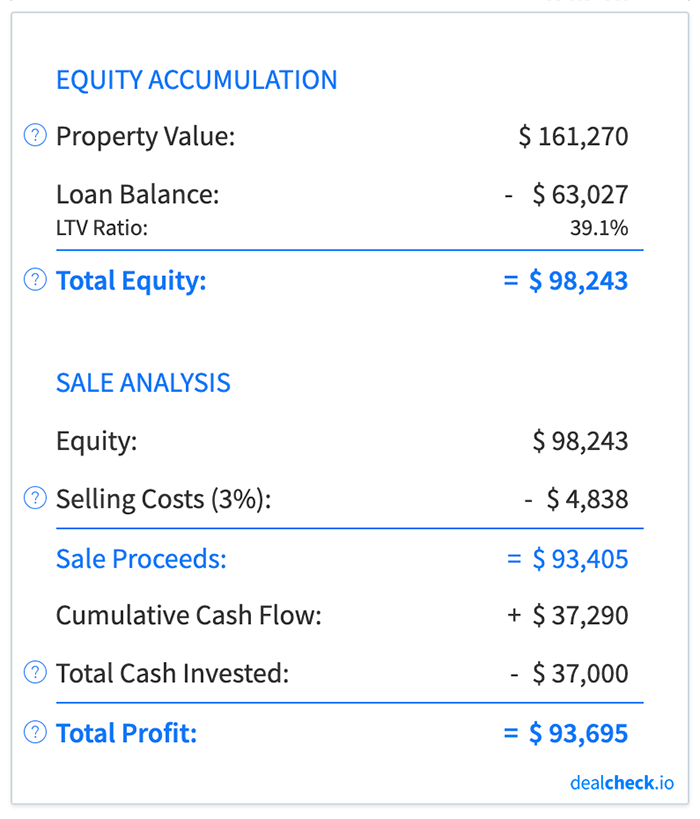Note: This article describes the ROI calculation specifically for rental properties, and not flips and rehab projects, which is calculated differently.

The return on investment, abbreviated as ROI, is one of the primary metrics of profitability and a measure of the return on invested capital used by investors when evaluating and comparing rental properties and BRRRR’s.

ROI is a rate of return that takes into account the cumulative cash flow, equity accumulation, and loan paydown and shows the total cumulative return on your invested capital if you were to sell the property at a given point in time.

Along with the internal rate of return (IRR), ROI is often used to compare the profitability of rental properties, as well as to compare the returns of rental properties to other asset classes, such as stocks, bonds, or commodities.

### The Return on Investment (ROI) Formula

The ROI for rental properties can be calculated by subtracting the total invested cash from the total profit from your investment, and dividing the result by the total invested cash:In the formula above, your total profit is comprised of your equity (property value minus the loan balance), less the closing costs at the time of sale, as well as the cumulative cash flow received during the time you owned the property.

The total invested cash represents all capital that was originally used to purchase and rehab the property. This usually includes the down payment on the loan (or the purchase price, if not using financing), purchase and closing costs, as well as rehab costs.

### Return on Investment Example

Let’s assume that you purchased a rental property for \$100,000 with a 20% down payment (\$20,000) and paid \$2,000 in closing costs. The home also needed \$15,000 of rehab work to make it rent-ready.

You owned the property for 10 years before selling it for about \$161,000 and paying \$4,800 in closing costs in the process. Throughout the 10 years, you also collected just over \$37,000 of cash flow from this property.

First, let’s calculate how much capital you spent to purchase and rehab this property – your total invested cash:

Total Invested Cash = Down Payment + Closing Costs + Rehab Costs = \$20,000 + \$2,000 + \$15,000 = \$37,000

Next, let’s calculate how much money you will receive when you sell this property after 10 years – your sale proceeds:

Sale Proceeds = Sale Price – Loan Balance – Closing Costs = \$161,270 – \$63,027 – \$4,838 = \$93,405Finally, let’s calculate your total return on investment after 10 years of ownership:

ROI = Sale Proceeds + Cumulative Cash Flow – Total Invested Cash / Total Invested Cash = \$93,405 + \$37,290 – \$37,000 / \$37,000 = 253%

So, what does this number tell you? It means that over a 10 year period, you received a 253% return on the original capital (\$37,000) you invested in this property. This is your total cumulative return after you liquidate your investment.

### Why ROI Can Be Negative During the First Few Years

Even if a rental property has positive cash flow, it is possible for its return on investment to be negative in the first few years of ownership.

The reason for this is that the ROI formula shown above assumes that the property is sold at the end of the specific period it is calculated for. And it takes into account not just the cash flow, but also price appreciation (or depreciation), selling costs, and loan paydown.

Once you account for these factors, it is possible that your cumulative cash flow in the first few years will not be enough to offset other costs, resulting in a total net loss if the property were to be sold. This is often the case if your initial purchase price was at or above the property’s market value, if the property value doesn’t appreciate, or if you will have high closing costs at the time of sale.

In fact, due to the compounding nature of ROI, you will often see it start at a relatively small figure and increase over time, as the price appreciation, loan paydown, and cumulative cash flow compound your return:So, while you may end up with a net loss (and a negative ROI) if you were to sell the property within the first few years of ownership, your net profit and ROI will grow over time as you continue to rent it out.

### Return on Investment vs. Internal Rate of Return

The internal rate of return (IRR) is another measure of profitability and return on invested capital used when evaluating rental properties. While the return on investment (ROI) shows the total cumulative return, the internal rate of return shows an average annualized return instead.

The ROI only shows the total return after a specific period of time and does not take into account the length of time it took to achieve this return.

For example, two properties may both have an ROI of 200%, but one may be able to achieve this in 5 years, while it will take 10 years for the other property to do the same. If you’re only looking at the ROI in isolation, you will miss this important distinction.

On the other hand, IRR is a time-sensitive metric that does take into account the time period you’re looking at. In the above example, the property that is able to achieve a 200% ROI in 5 years will have a higher IRR than the other property, which may indicate a better-performing asset.

Because of this, it is often helpful to consider both the ROI and IRR together when evaluating and comparing investment properties. You can use the ROI to compare the total returns on your capital, and IRR to compare the average annualized versions of the same.

### Calculate ROI and Dozens of Other Metrics in Seconds

The DealCheck property analysis app makes it easy to calculate the return on investment, along with dozens of other property analysis metrics for rental properties and BRRRR’s in seconds.